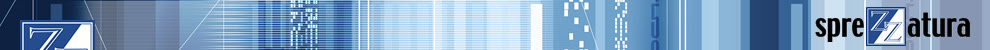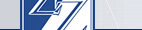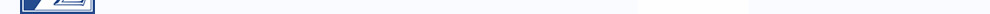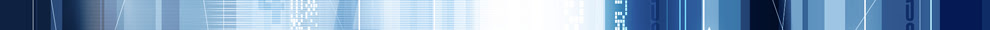By apk | Monday, 4 February 2019 15:34 | 0 Comments
A recent posting on the Rev forum asked about using compound assignment operators with dynamic arrays on the left side of the operator.  A compound assignment operator is an operator that does multiple actions with one command.  The compound assignment operators in Basic+ are
-= (subtract and assign)
:= (concatenation and assign)
Specifically, the poster was asking why the statement

workRec<10> += CHECK_HISTORY<CHECK.AMOUNT\$>

would not compile.

It's been a long time since I looked at the meta for this( 1994-95) with the goal of allowing this structure in ARev 3.12, but I'll tell you what I remember.

Without going into detail on how the compiler works, it parses the source and turns each command into a set of opcodes.  Opcodes are more or less stored in a Reverse Polish notation structure.  So, the command

= j

becomes

i, j, = <eoe>

(where <eoe> means "end of expression")

This means, when the engine processes the opcodes, it puts the contents of "i" on the stack, then the contents of "j" on the stack, then it reads the "=", so assigns the contents of "j" to "i".  Pretty simple.

Operator/concatenation operators (+=, -=, :=) are a form of "syntactic sugar".  However, the important point is that the angle brackets are also syntactic sugar.  Angle brackets are actually the "replace" and "extract" commands.  When you're trying to compile "i<j> += k" basically you're ending up with sugar sugar (oh, honey, honey).

When the angle brackets are used on the left side of an operator, the compiler parses through the source text and then generates opcodes to match the "replace" command.  This isn't a precompiler, where equates are literally placed into the source before compilation.  The system parses the source text and then puts in the opcodes as if you had entered a replace command.  Taking a simpler statement

i<j> = k

what ends up being compiled is the same as if you typed

= replace( i, j, 0, 0, k)

Reversing the statement

= i<j>

ends up as

= extract( i, j, 0, 0 )

The compiler runs through a different set of rules and instructions, but the output is identical.  Decompiled code will always output extract and replace commands, because there isn't a functional opcode for dynamic arrays.  The object code for the two lines should be exactly the same.

The operator/concatenation functions are similar.  There aren't compiler operators for these functions.  The compiler just generates the outputs as if you typed in the full command.  So, the statement

i += k

ends up being

= i + k

So, going back to our stack example, "i += k" ends up on the stack as

i, k + = <eoe>

What does all this mean in terms of your specific example?  When compiled, the statement

workRec<10> += CHECK_HISTORY<CHECK.AMOUNT\$>

ends up being treated by the compiler as

workRec = replace( workRec, 10, 0, 0, (extract( workRec, 10, 0, 0 ) |
+ extract(check_history,12,0,0)))

The Basic+ compiler is essentially a rules based engine, and works on the idea of

expression operator expression

The "sugar" works because the compiler parses the statement and pushes the desired opcodes into the object stack.  When putting "sugar" on "sugar", the first "sugar" is pushed onto the object stack before it can get to the second "sugar", and the system isn't prepared for a second set of manipulations, since the original expression is closed.

The other part of the equation here is that Basic+ supports two types of arrays, dynamic and dimensioned.  Dynamic arrays are arrays accessed by the angle bracket " i<j> " while dimensioned arrays require the "dim" statement and are accessed using parentheses " i(j) ".  Each element in a dimensioned array is a distinct variable.  So i(1and  i(2are different variables.  Dynamic arrays are more of a logical and syntactical concept.  The main elements (1st dimension, if you will) are just delimited by characters (\FE\,  char(254) or @FM).  The extract, replace and insert statements understand the structure, so it becomes a logical array.

In order to work on a single element of a dynamic array, it needs to be extracted.  Otherwise, it's really just an offset into a portion of a longer string type variable.  So, for a fuller example of what

workRec<10> += CHECK_HISTORY<CHECK.AMOUNT\$>

compiles down to, we really have something more like

extract( workRec, 10, 0, 0 ) += extract( check_history, 12, 0, 0 )

and you can't have extract on the left side of an operator.

For more information on the the compiler, see our previous posting All Things Being Equals.

Labels: , , ,